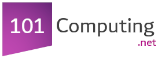```1.  #Higher or Lower Game - www.101computing.net/higher-or-lower/
2.  from random import randint
3.
4.  #Initialise the game
5.  number=randint(1,1000)
6.  score=0
7.
8.  while True:
9.    print("Current Number: " + str(number))

10.   #Collect user guess
11.   userGUess = input("Will the next number be higher (type +) or lower (type -)?")
12.
13.   #Generate next number
14.   nextNumber=randint (1,1000)
15.   print("Next number is: " + str(nextNumber))
16.
17.   #Check if the user is correct
18.   if userGUess=="+" and nextNumber >= number:
19.       score+=1
20.       number=nextNumber
21.   elif userGUess=="-" and nextNumber <= number:
22.       score+=1
23.       number=nextNumber
24.   else:
25.       print "game over"
26.       break
27.
28.   #Display score so far
29.   print ("Your score is: " + str(score))
30.
```
Score: